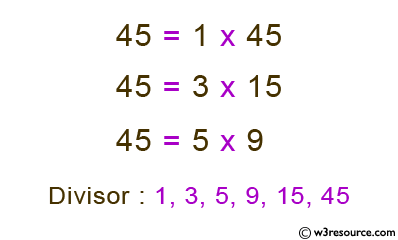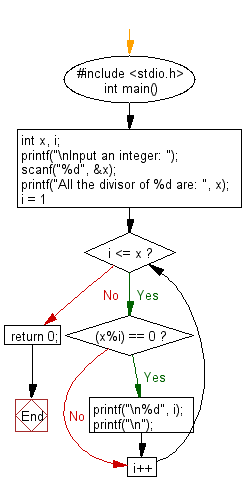﻿ C Program: Read an integer and find all its divisor - w3resource

# C Exercises: Read an integer and find all its divisor

## C Basic Declarations and Expressions: Exercise-47 with Solution

Write a C program that finds all the divisors of an integer.

Pictorial Presentation:Sample Solution:

C Code:

``````#include <stdio.h>
int main() {
int x, i;

// Get an integer input from the user
printf("\nInput an integer: ");
scanf("%d", &x);

// Print all the divisors of x
printf("All the divisors of %d are: ", x);
for(i = 1; i <= x; i++) {
if((x%i) == 0){
printf("\n%d", i);
printf("\n");
}
}

return 0;
}
``````

Sample Output:

```Input an integer: 45
All the divisor of 45 are:
1

3

5

9

15

45
```

Flowchart:C programming Code Editor:

What is the difficulty level of this exercise?

Test your Programming skills with w3resource's quiz.

﻿